# Possible combinations - word

How many ways can the letters F, A, I, R be arranged?

Correct result:

n =  24

#### Solution:

$n=4!=24$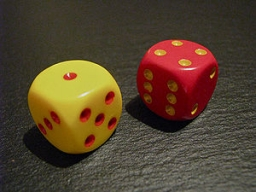We would be pleased if you find an error in the word problem, spelling mistakes, or inaccuracies and send it to us. Thank you!Tips to related online calculators
Would you like to compute count of combinations?

## Next similar math problems:

• Alopecia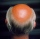Medical literature indicates that 45% of men suffer from alopecia. For random sample of 8 men, calculate the probability that: (a) exactly four men suffer from alopecia. (b) at most two men suffer from alopecia.
• STRESSED wordEach letter in STRESSED is printed on identical cards, one letter per card, and assembled in random order. Calculate the probability that the cards spell DESSERTS when assembled.
• ClassroomOf the 26 pupils in the classroom, 12 boys and 14 girls, four representatives are picked to the odds of being: a) all the girls b) three girls and one boy c) there will be at least two boys
• Boys and girlsThere are eight boys and nine girls in the class. There were six children on the trip from this class. What is the probability that left a) only boys b) just two boys
• SalamiHow many ways can we choose 5 pcs of salami if we have 6 types of salami for 10 pieces and one type for 4 pieces?
• Wagons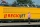We have six wagons, two white, two blue, and two red. We assemble trains from them, wagons of the same color are exactly the same, so if we change only two white wagons on a train, it's still the same train, because I don't know any different. How many diHow many ways can we thread 4 red, 5 blue, and 6 yellow beads onto a thread?
• Two groupsThe group of 10 girls should be divided into two groups with at least 4 girls in each group. How many ways can this be done?There are 15 boys and 12 girls at the graduation party. Determine how many four couples can be selected.
• Three workplacesHow many ways can we divide nine workers into three workplaces if they need four workers in the first workplace, 3 in the second workplace and 2 in the third?
• Boys and girlsThere are 11 boys and 18 girls in the classroom. Three pupils will answer. What is the probability that two boys will be among them?
• Families 2Seven hundred twenty-nine families are having six children each. The probability of a girl is 1/3, and the probability of a boy is 2/3. Find the number of families having two girls and four boys.
• Tournament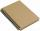Six teams entered the basketball tournament. How many matches will be played if each team has to play one match with each other?
• Word MATEMATIKAHow many words can be created from the phrase MATEMATIKA by changing the letters' order, regardless of whether the words are meaningful?
• Sum or productWhat is the probability that two dice fall will have the sum 7 or product 12?
• Three reds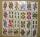What is the probability that when choosing 3 carats from seven carats, all 3 reds will be red?
• CardsFrom a set of 32 cards we randomly pull out three cards. What is the probability that it will be seven king and ace?